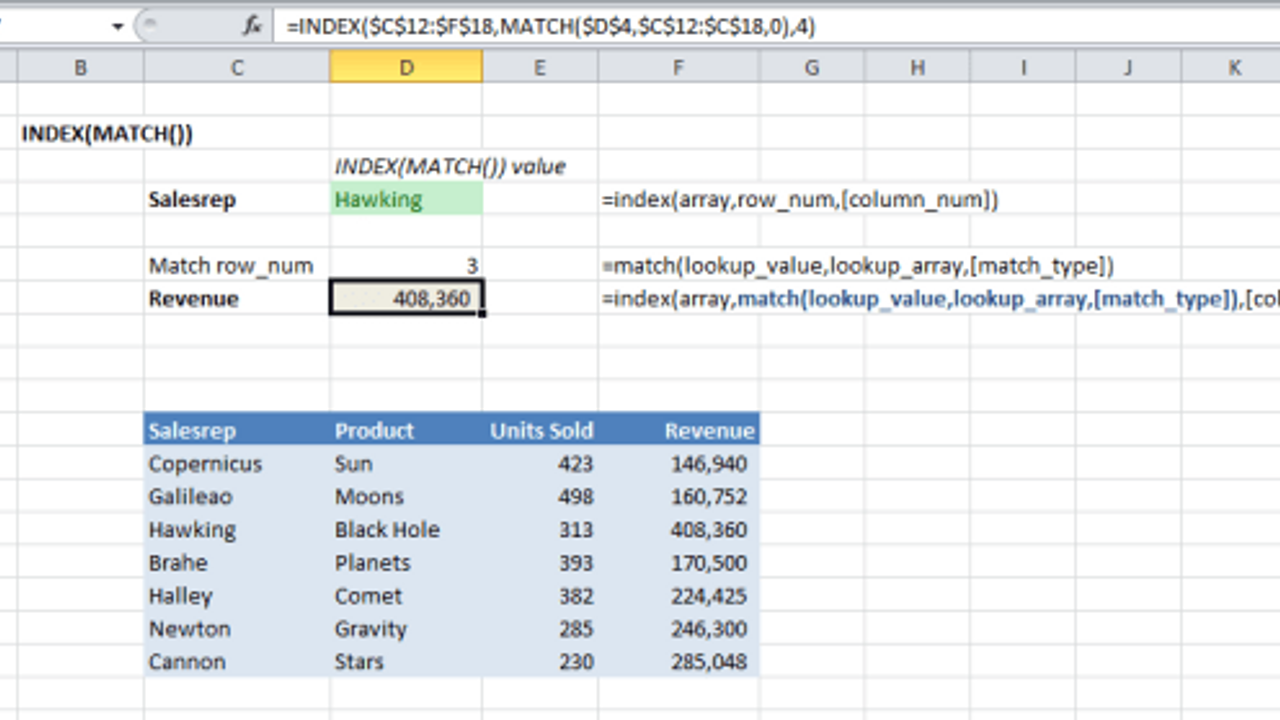# Using INDEX and MATCH to Retrieve Excel DataConsultants and data analysts need to be able to find the exact data they need to analyze client data. Usually these large tables or lists of data come from large databases and are imported into Excel. But, once in Excel how can you search and find the exact data you need?

Use INDEX and MATCH to retrieve data from an Excel table when you want the greatest flexibility and speed in retrieving data. INDEX and MATCH takes a little more work to create than VLOOKUP, but it is far more flexible and powerful. It also enables you to animate charts.

MATCH searches down any column for the row containing matching content. INDEX then looks left or right in that row to retrieve data from the column you specify.

One of the most powerful features of INDEX and MATCH is that the two functions work together to extract data from anywhere in a table. You aren’t limited to looking for a matching word in the leftmost column. The MATCH function looks down any column to find the row containing the data you are searching for and then the INDEX function retrieves data from any column in that row. INDEX and MATCH do away with VLOOKUP’s limitation of using only the leftmost column for the matching word.

Another powerful feature of INDEX and MATCH is in animating charts. MATCH finds an initial piece of data in a table. Then, by adding an incremental number to row-num and col_num in INDEX, your charts can animate or scroll through data in the table. To animate data in a chart, use a spin button or scrolling bar to add an increment to the row_num or col_num retrieved and charted.

Download the example and tutorial files for learning INDEX and MATCH functions

The INDEX function has this syntax,

=INDEX(array,row_num,[column_num])

array                                             is the range containing data.

row_num                                    is the row containing the data.

[column_num]                          is the column number containing the data to be retrieved.

The MATCH function looks through data to find a match for the value you are looking for. It can look down a column or across a row. In most cases you will use MATCH to look down any column in the table. (Unlike VLOOKUP, MATCH works on any column in the table. VLOOKUP only looks at the leftmost column.) The MATCH function looks like this,

=MATCH(lookup_value,lookup_array,[match_type])

lookup_value                           is the value being searched for.

lookup_array                            is the column or row containing the data.

[match_type]                            is one of three values; 1 is less than a match, 0 is an exact match,                                                     and -1 is greater than a match

You can probably already see how these two work together. MATCH finds the row containing the data and then INDEX looks left or right across the row to retrieve the data a column in that row.

When used together they look like this,

=INDEX(array,MATCH(lookup_value,lookup_array,[match_type]),[column_num])

The functions used in the example are,

=INDEX(\$C\$12:\$F\$18,MATCH(\$D\$4,\$C\$12:\$C\$18,0),4)

MATCH uses “Hawking” in \$D\$4 to search through \$C\$12:\$C\$18. The 0 as the last parameter specifies that MATCH will only find exact matches. In this example the result is row 3 as shown in cell D6. (The result in D6 isn’t used in the INDEX formula. It is only there for you to examine in the downloadable example.) INDEX retrieves the result from the third (3) row and the fourth (4) column in the range \$C\$12:\$F\$18.

In most cases you will use MATCH to look down a column, but it can be used to look down any column or across any row to find a match. This makes a very powerful combination for two-way lookups where the user can lookup matching data in a column and then extract data from any row they choose.

Download the example and tutorial files for learning INDEX and MATCH functions